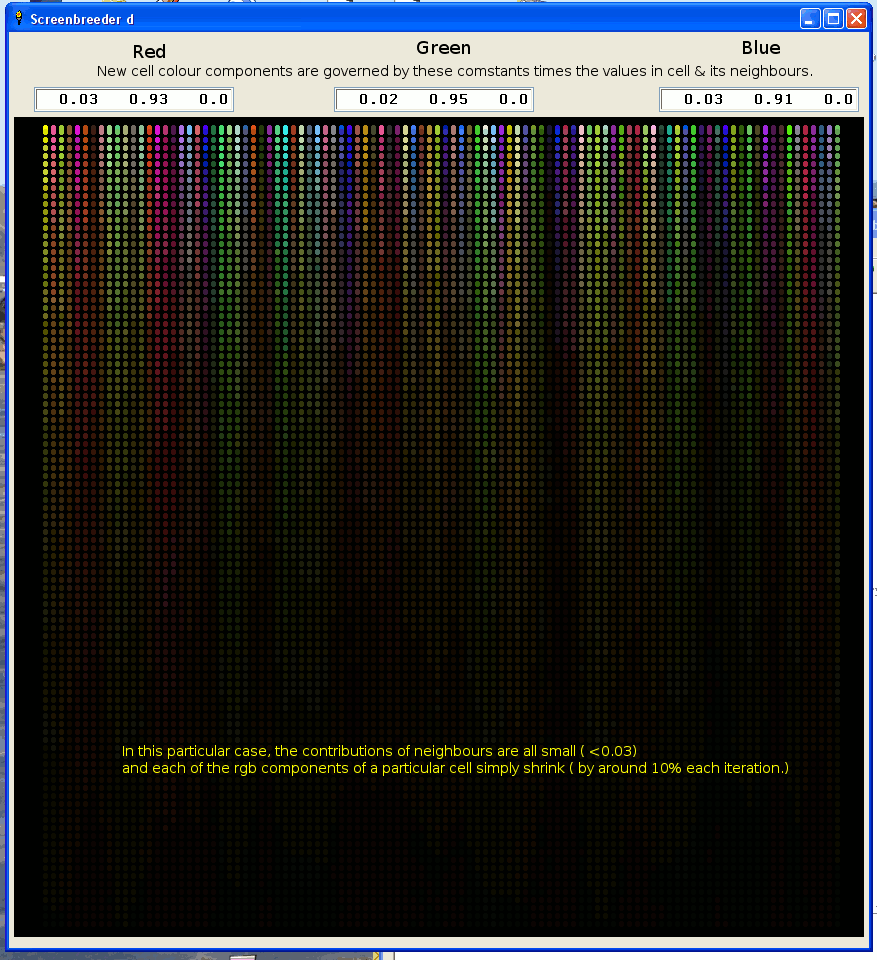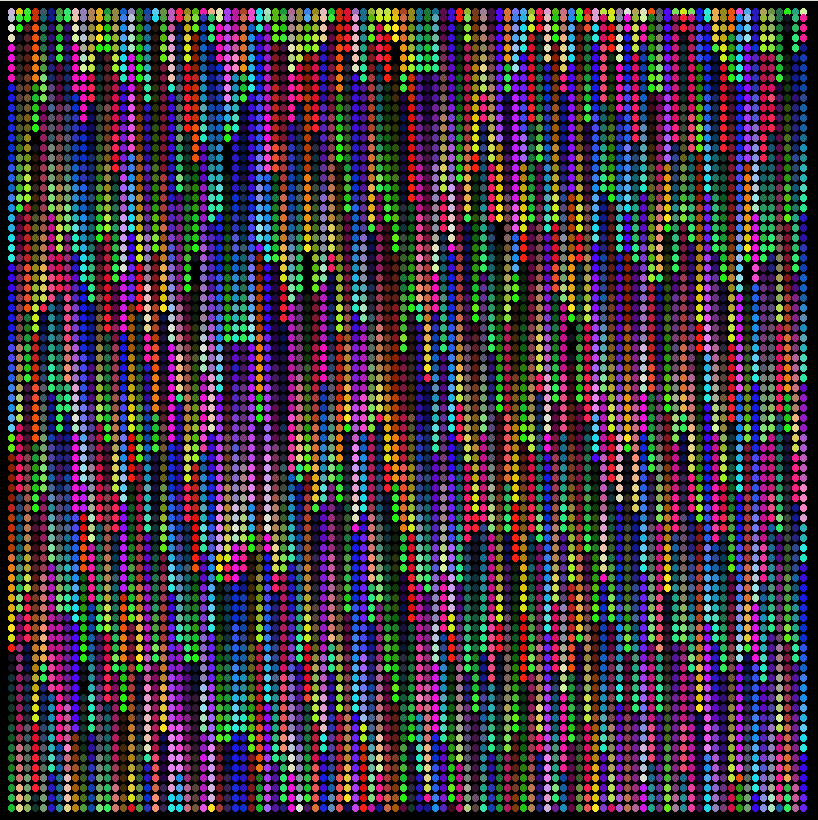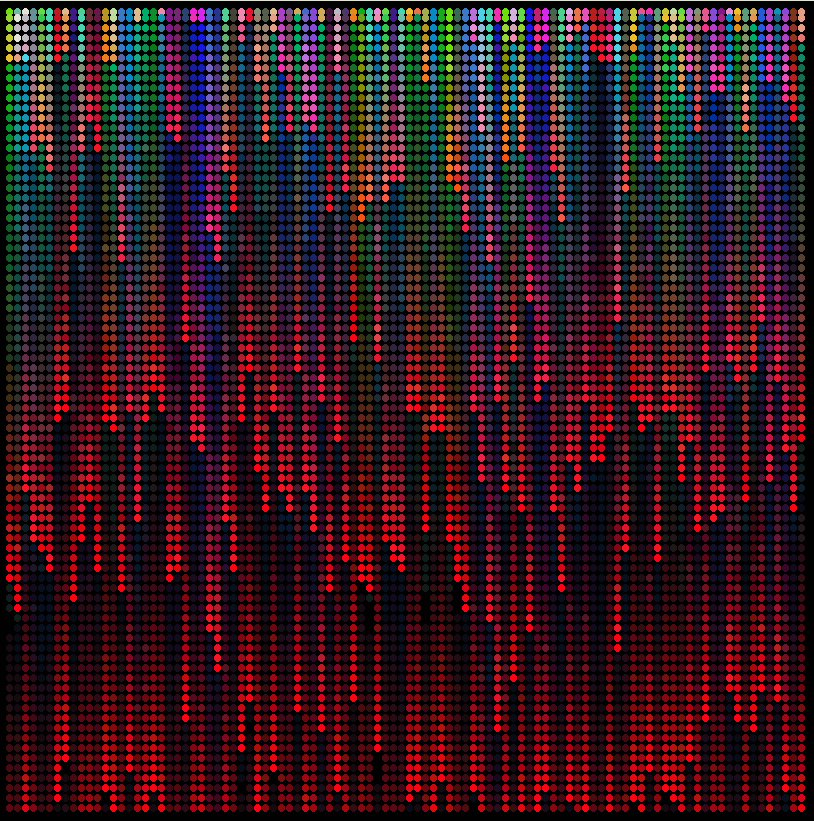# 1D evolution

Imagine a one-dimensional ( '1D') row of 100 cells, each holding a random initial colour. Draw as a screen row.Now take a fraction of the r g & b colour components of any given cell & its immediate neighbours ( assumed to 'wrap round' so the end cells join up).

Hold these in temporary storage 'til the row is complete, then replace the original cells and draw one place down the screen. You will get patterns of various types depending on the constants used.

You can think of the 9 constants as 'gene'. A different gene-set is used throughout any one run. For testing, include the 'randomize' line, to get the same initial row every time, and if you leave as-is, the same gene.

You can of course enter your own gene as 9 constants rather than have the program generate them.

Better still, make programming mistakes like I did & see what serendipity creates!!

Typical results follow.```
'   Screenbreeder_e.bas

nomainwin

UpperLeftX   = 10
UpperLeftY   = 10
WindowWidth  =868
WindowHeight =750

graphicbox #w.g,   5, 85, 850, 820
textbox    #w.t1, 25, 55, 200,  25
textbox    #w.t2,325, 55, 200,  25
textbox    #w.t3,650, 55, 200,  25

dim row( 100, 3), temp( 100, 3)
global ar, br, cr,   ag, bg, cg,      ab, gb, bb

'   REM out the following line to get different results every time
'   randomize 0.5

open "Screenbreeder d" for window as #w

#w       "trapclose [quit]"
#w.g     "down ; size 5 ; fill black"
#w.t1    "!font courier 14 bold"
#w.t2    "!font courier 14 bold"
#w.t3    "!font courier 14 bold"

for x =0 to 99
row( x, 1) =int( 256 *rnd( 1))
row( x, 2) =int( 256 *rnd( 1))
row( x, 3) =int( 256 *rnd( 1))
#w.g "color "; row( x, 1); " "; row( x, 2); " "; row( x, 3)
#w.g "set "; 30 +x *8; " "; 10
scan
next x

'   The following constants decide how much the previous cell & its neighbours
'       contribute to the new cell.
'   Think of them as 9 'genes' which determine the pattern.

'   At present set so close to previous colour, with minor neighbour contributions.

ar =rnd( 1) /5   /5: br =0.9 +rnd( 1) /5: cr =rnd( 1) /5   /5
ag =rnd( 1) /5   /5: bg =0.9 +rnd( 1) /5: cg =rnd( 1) /5   /5
ab =rnd( 1) /5   /5: bb =0.9 +rnd( 1) /5: cb =rnd( 1) /5   /5

#w.t1 "  "; using( "#.##", ar); "   "; using( "#.##", br); "   "; using( "#.##", cr)
#w.t2 "  "; using( "#.##", ag); "   "; using( "#.##", bg); "   "; using( "#.##", cg)
#w.t3 "  "; using( "#.##", ab); "   "; using( "#.##", bb); "   "; using( "#.##", cb)

for y =1 to 100
call breed
for x =0 to 99
#w.g "color "; row( x, 1); " "; row( x, 2); " "; row( x, 3)
#w.g "set "; 30 +x *8; " "; 8 *y +6
scan
next x
#w.g "flush"
next y

wait

sub breed
for x =0 to 99
'   change each colour attribute depending on its previous value, and neighbours
temp( x, 1) =max( 0, ( ar *row( ( x +99) mod 100, 1) +br *row( x, 1) +cr *row( ( x +1) mod 100, 1) /3) mod 256)
temp( x, 2) =max( 0, ( ag *row( ( x +99) mod 100, 2) +bg *row( x, 2) +cg *row( ( x +1) mod 100, 2) /3) mod 256)
temp( x, 3) =max( 0, ( ab *row( ( x +99) mod 100, 3) +bb *row( x, 3) +cb *row( ( x +1) mod 100, 3) /3) mod 256)
next x
for x =0 to 99
row( x, 1) =temp( x, 1)
row( x, 2) =temp( x, 2)
row( x, 3) =temp( x, 3)
next x
end sub

[quit]
close #w
end
```